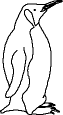EnchantedLearning.com is a user-supported site.
As a bonus, site members have access to a banner-ad-free version of the site, with print-friendly pages.

 Go to the AnswersMath Pages EnchantedLearning.com Divide Eight Birds Divide the birds into groups to do division. Visual Division PagesDivision Pages

 Divide the penguins into two equal groups.How many penguins are there? How many groups of penguins are there? How many penguins are in each group?
8 ÷ 2 =

 Divide the eagles into four equal groups.How many eagles are there? How many groups of eagles are there? How many eagles are in each group?
8 ÷ 4 =

 Divide the owls into eight equal groups.How many owls are there? How many groups of owls are there? How many owls are in each group?
8 ÷ 8 =

## Enchanted Learning Search

 Search the Enchanted Learning website for: